# 基于飞桨复现强化学习进阶算法SAC，让月球着陆器顺利着陆

2020/10/14 16:47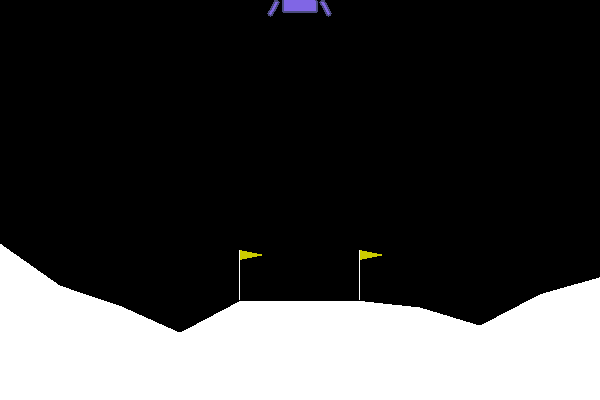• SAC算法论文简介

• SAC算法样例代码简介

• 介绍如何用SAC算法玩转月球着陆器

下载安装命令

## CPU版本安装命令

## GPU版本安装命令
pip install -f https://paddlepaddle.org.cn/pip/oschina/gpu paddlepaddle-gpu

### SAC算法论文

SAC是Soft Actor-Critic的缩写，由伯克利人工智能研究实验室(BAIR)的Tuomas Haarnoja等人，提出于2018年。原文链接：

https://arxiv.org/abs/1801.01290

• 非常高的采样复杂度（very high sample complexity）

• 脆弱的收敛性质（brittle convergence properties）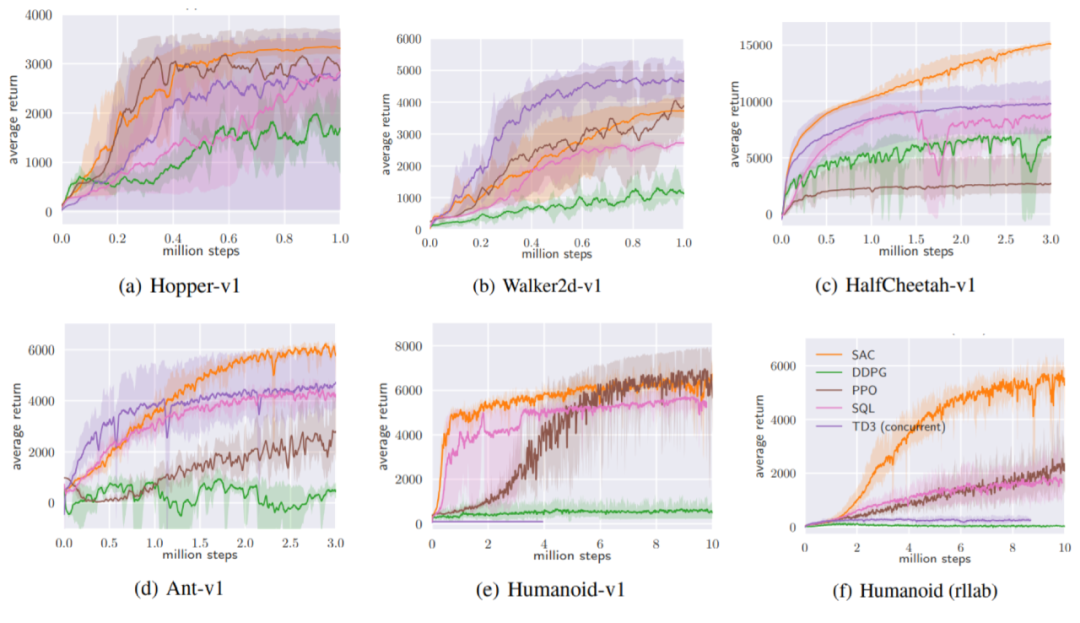### SAC算法样例

（GitHub访问困难的同学可以自己搜索一下Gitee，也可以在example目录下找到的）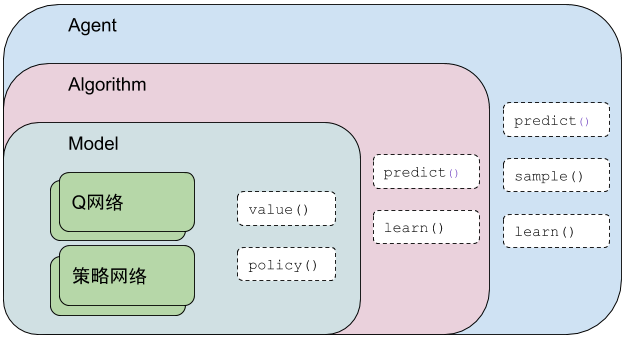PARL框架的结构大致如上图所示，采用了层层嵌套的结构，适用于绝大多数强化学习算法。具体到SAC算法的话，最内层Model封装了Q网络与策略网络的网络结构，通过value()与policy()两个方法输出Q值和动作值。向外一层，Algorithm主要封装了损失函数，通过predict()向外输出动作值，通过learn()向内更新Model的网络权重。最外层的Agent主要负责与环境的交互并把从环境得到的数据喂给Algorithm。

• mujoco_agent.py

• mujoco_model.py

• train.py

class ActorModel(parl.Model):
def __init__(self, act_dim):
hid1_size = 400
hid2_size = 300
self.fc1 = layers.fc(size=hid1_size, act='relu')
self.fc2 = layers.fc(size=hid2_size, act='relu')
self.mean_linear = layers.fc(size=act_dim)
self.log_std_linear = layers.fc(size=act_dim)
def policy(self, obs):
hid1 = self.fc1(obs)
hid2 = self.fc2(hid1)
means = self.mean_linear(hid2)
log_std = self.log_std_linear(hid2)
log_std = layers.clip(log_std, min=LOG_SIG_MIN, max=LOG_SIG_MAX)
return means, log_std


CriticModel中定义了Critic的网络结构（Q网络）并且实现了value方法。这个方法根据输入obs和策略网络输出的动作值，通过内部的Q网络，来输出Q值。

class CriticModel(parl.Model):
def __init__(self):
hid1_size = 400
hid2_size = 300
self.fc1 = layers.fc(size=hid1_size, act='relu')
self.fc2 = layers.fc(size=hid2_size, act='relu')
self.fc3 = layers.fc(size=1, act=None)
self.fc4 = layers.fc(size=hid1_size, act='relu')
self.fc5 = layers.fc(size=hid2_size, act='relu')
self.fc6 = layers.fc(size=1, act=None)
def value(self, obs, act):
hid1 = self.fc1(obs)
concat1 = layers.concat([hid1, act], axis=1)
Q1 = self.fc2(concat1)
Q1 = self.fc3(Q1)
Q1 = layers.squeeze(Q1, axes=)
hid2 = self.fc4(obs)
concat2 = layers.concat([hid2, act], axis=1)
Q2 = self.fc5(concat2)
Q2 = self.fc6(Q2)
Q2 = layers.squeeze(Q2, axes=)
return Q1, Q2


class MujocoAgent(parl.Agent):
def predict(self, obs):
obs = np.expand_dims(obs, axis=0)
act = self.fluid_executor.run(
self.pred_program, feed={'obs': obs},
fetch_list=[self.pred_act])
return act

def sample(self, obs):
obs = np.expand_dims(obs, axis=0)
act = self.fluid_executor.run(
self.sample_program,
feed={'obs': obs},
fetch_list=[self.sample_act])
return act

def learn(self, obs, act, reward, next_obs, terminal):
feed = {
'obs': obs,
'act': act,
'reward': reward,
'next_obs': next_obs,
'terminal': terminal
}
[critic_cost, actor_cost] = self.fluid_executor.run(
self.learn_program,
feed=feed,
fetch_list=[self.critic_cost, self.actor_cost])
self.alg.sync_target()
return critic_cost, actor_cost



    actor = ActorModel(act_dim)
critic = CriticModel()
algorithm = parl.algorithms.SAC(
actor,
critic,
max_action=max_action,
gamma=GAMMA,
tau=TAU,
actor_lr=ACTOR_LR,
critic_lr=CRITIC_LR)
agent = MujocoAgent(algorithm, obs_dim, act_dim)



    from parl.utils import ReplayMemory
rpm = ReplayMemory(MEMORY_SIZE, obs_dim, act_dim)



### SAC算法实践

import gym



env = gym.make('LunarLanderContinuous-v2')



np.save('train_step_list', train_step_list)
np.save('train_reward_list', train_reward_list)
np.save('evaluate_step_list', evaluate_step_list)
np.save('evaluate_reward_list', evaluate_reward_list)



train_step_list = np.load('train_step_list.npy')

plt.figure()
plt.title('train reward')
plt.xlabel('step')
plt.ylabel('reward')
plt.plot(train_step_list, train_reward_list)
plt.grid()
plt.show()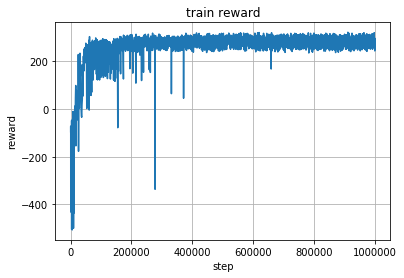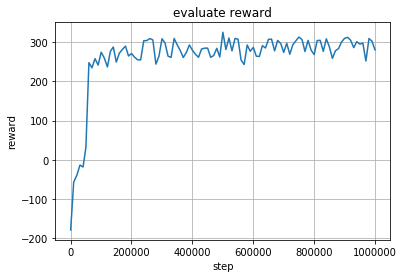### 全文回顾

https://aistudio.baidu.com/aistudio/projectdetail/888258

下载安装命令

## CPU版本安装命令

## GPU版本安装命令


0
0 收藏

### 作者的其它热门文章0 评论
0 收藏
0